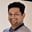Related Tags

java
array
communitycreator

# How to print multi-dimensional arrays in JavaTarun Telang

In Java, printing an array is a common task. Although, outputting the content of a one-dimensional array is straightforward enough, doing it for the content of a multi-dimensional array is more complex.

To print a multi-dimensional array, you first need to convert its content to a String using nested loops. The code below prints arrays of different dimensions.

class Main {
public static void main( String args[] ) {

// 1-D array
int[] arr = {0, 1, 2, 3, 4};

// 2-D array
int[][] matrix = {
{0, 1, 2}, {2, 3, 4}, {5, 6, 7}
};

// 3-D array
int[][][] multiDimArray= {
{
{0, 1}, {2, 3}, {3, 4}
},
{
{5, 6}, {7, 8}, {8, 9}
}
};

System.out.print("1-D Array: \n[");
// printing a 1-D array using loop
for (int n: arr) {
System.out.print(n + " "); // printing each item
}
System.out.println("]\n");

System.out.print("2-D Array: \n[");
// printing a 2-D array using two nested loops
for (int[] array: matrix) {
System.out.print("[");
for (int n: array) {
System.out.print(n + " "); // printing each item
}
System.out.print("]"); // printing new line
}
System.out.println("]\n");

System.out.println("3-D Array: ");
// printing a 3-D array using three nested loops
for (int[][] m: multiDimArray) {
System.out.print("[");
for (int[] a: m) {
System.out.print("[");
for (int n: a) {
System.out.print(n + " "); // printing each item
}
System.out.print("]");
}
System.out.print("]");
}
System.out.println("]");

}
}

We need to use the number of nested loops to iterate on each item of an N-dimensional array before printing it. As the number of dimensions increases, the code becomes more complex and harder to maintain.

However, there is a cleaner approach for this using the Arrays.toString() and Arrays.deepToString() library methods defined within the java.util.Arrays class.

To print a one-dimensional array, you need to call the Arrays.toString() method and pass the array as an argument. However, this only works with one-dimensional arrays.

To print a multi-dimensional array, you instead need to call the Arrays.deepToString() method and pass the multi-dimensional array as an argument.

See the code example below for more clarity on this:

import java.util.Arrays;
class Main {
public static void main( String args[] ) {

// 1-D array
int[] arr = {0, 1, 2, 3, 4};

// 2-D array
int[][] matrix = {{0, 1, 2}, {2, 3, 4}, {5, 6, 7}};

// 3-D array
int[][][] multiDimArray= {
{
{0, 1}, {2, 3}, {3, 4}
},
{
{5, 6}, {7, 8}, {8, 9}
}
};

System.out.println("1-D Array: ");
// printing a 1-D array using Arrays.toString
System.out.println(Arrays.toString(arr));

System.out.println("\n2-D Array: ");
// printing a 2-D array using Arrays.deepToString
System.out.println(Arrays.deepToString(matrix));

System.out.println("\n3-D Array: ");
// printing a 3-D array using Arrays.deepToString
System.out.println(Arrays.deepToString(multiDimArray));

}
}

## Conclusion

You cannot print an array in Java just by using System.out.println(). Instead, you need to loop through each item and convert it to String before printing it.

A cleaner approach would be to use the appropriate utility method from the java.util.Arrays class. To print the content of a one-dimensional array, use the Arrays.toString() method. To print the content of a a multi-dimensional array, use the Arrays.deepToString() method.

RELATED TAGS

java
array
communitycreator

CONTRIBUTORTarun Telang
RELATED COURSES

View all Courses

Keep Exploring

Learn in-demand tech skills in half the time